## 2.24Summary of tensor algebra

Below is tensor algebra applied to Cartesian (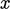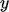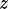) co-ordinates using: scalar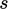; vectors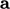,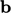; tensors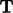,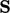,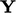.

### Products

• Inner product of two vectors, Sec. 2.3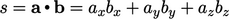• Outer product of two vectors, Sec. 2.8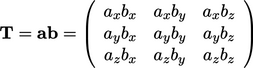• Inner product of vector and tensor, Sec. 2.6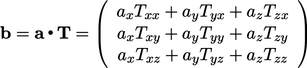• Inner product of two tensors, Sec. 2.8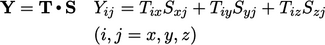• Double inner product of two tensors, Sec. 2.17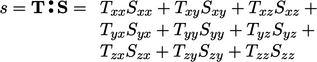• Cross product of two vectors, ﬁrst used in Sec. 3.3 , produces a vector with components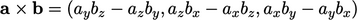(2.70)

### Tensors and operations

• Transpose of a tensor, Sec. 2.7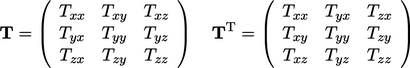• Symmetric and skew tensors, Sec. 2.7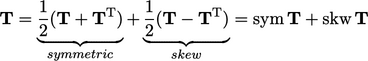• Trace of a tensor, Sec. 2.10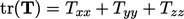• Identity tensor, Sec. 2.8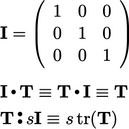• Deviatoric and spherical tensors, Sec. 2.10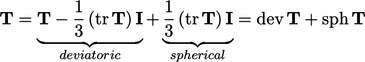Notes on CFD: General Principles - 2.24 Summary of tensor algebra